# Reason + natural numbers - math problems

#### Number of problems found: 238

• We rollWe roll two dice A. - what is the probability that the sum of the falling numbers is at most 4 B. - is at least 10 C. - is divisible by 5?
• We bought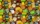We bought 140 fruit, 196 chocolate and 84 caramel lollipops. How many of the same packages can we prepare from them?
• THE FARM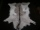Hens, goats, and 3 cows grow on the farm. All animals have a total of 19 heads and 56 legs. How many goats live on the farm, and how many hens live on the farm?
• Harry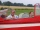Harry Thomson bought a large land in the shape of a rectangle with a circumference of 90 meters. He divided it into three rectangular plots. The shorter side has all three plots of equal length, their longer sides are three consecutive natural numbers. Fi
• Three-digit numbers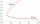How many three-digit numbers are not closer to 600 on the number axis than to 400?
• Five-digit numberAnna thinks of a five-digit number that is not divisible by three or four. If he increments each digit by one, it gets a five-digit number that is divisible by three. If he reduces each digit by one, he gets a five-digit number divisible by four. If it sw
• The difference 2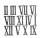The difference between the two numbers is 25. The smaller number is 1/6th of the larger number. What is the value of the smaller number?
• Pigs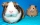A buyer said, "I want to buy 100 pigs for 100 denarii. An adult pig costs 10 denarii, a sow 5 denarii, two little piglets are worth 1 denarius. " How many pigs, sows and piglets could he buy for exactly 100 denarii?
• TogetherThe three friends divided the balls in a ratio of 6: 5: 4. Some two of them got a total of 126 balls. How many balls were there together?
• Tourists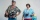Tourists hire a guide. Everyone will pay the same amount. If there are two less of them, they will pay 14 crowns more, and if there are three more, they will pay 14 crowns less. How many tourists are there and how much have the guides paid.
• Karolína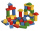Karolína chose 5 bodies from the kit - white, blue and gray cubes, a blue cylinder and a white triangular prism. How many different roof towers can be built one by one if all the blue bodies (cube and cylinder) are not placed on top of each other?
• Two grandmothersTwo grandmothers went to sell eggs at the market, and they had a total of 100. When they sold all the eggs, they made the same money. The first grandmother says to the second: "If I sold my eggs for your price, I would earn 15 crowns. " The other grandmot
• Cups on the shelf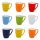Two green, three red, and two yellow cups should be placed side by side on the shelf. a) How many different ways of setting up can arise? b) How many different ways of arranging can arise if cups of the same color stand side by side?
• Coloured numbersMussel wrote four different natural numbers with coloured markers: red, blue, green and yellow. When the red number divides by blue, it gets the green number as an incomplete proportion, and yellow represents the remainder after this division. When it div
• Squirrels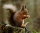The squirrels discovered a bush with hazelnuts. The first squirrel plucked one nut, the second squirrel two nuts, the third squirrel three nuts. Each new squirrel always tore one nut more than the previous squirrel. When they plucked all the nuts from the
• Six-eightsSix-eights of the one hundred pupils joined the Math Glee club. If the Math Glee club members were grouped into three, how many members were in each group?
• GranddaughterIn 2014, the sum of the ages of Meghan's aunt, her daughter and her granddaughter was equal to 100 years. In what year was the granddaughter born, if we know that the age of each can be expressed as the power of two?
• Shepherd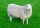Kuba makes a deal with a shepherd to take care of his sheep. Shepherd said to Kuba that he would receive twenty gold coins and one sheep after a year of service. But Kuba resigned just after the seventh month of service. But shepherd rewarded him and paid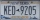A license plate has 3 letters followed by 4 numbers. Repeats are not allowed for the letters, but they are for the numbers. If they are issued at random, what is the probability that the 3 letters are in alphabetical order and the 3 numbers are consecutiv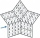Determine all pairs (m, n) of natural numbers for which is true: m s (n) = n s (m) = 70, where s (a) denotes the digit sum of the natural number a.